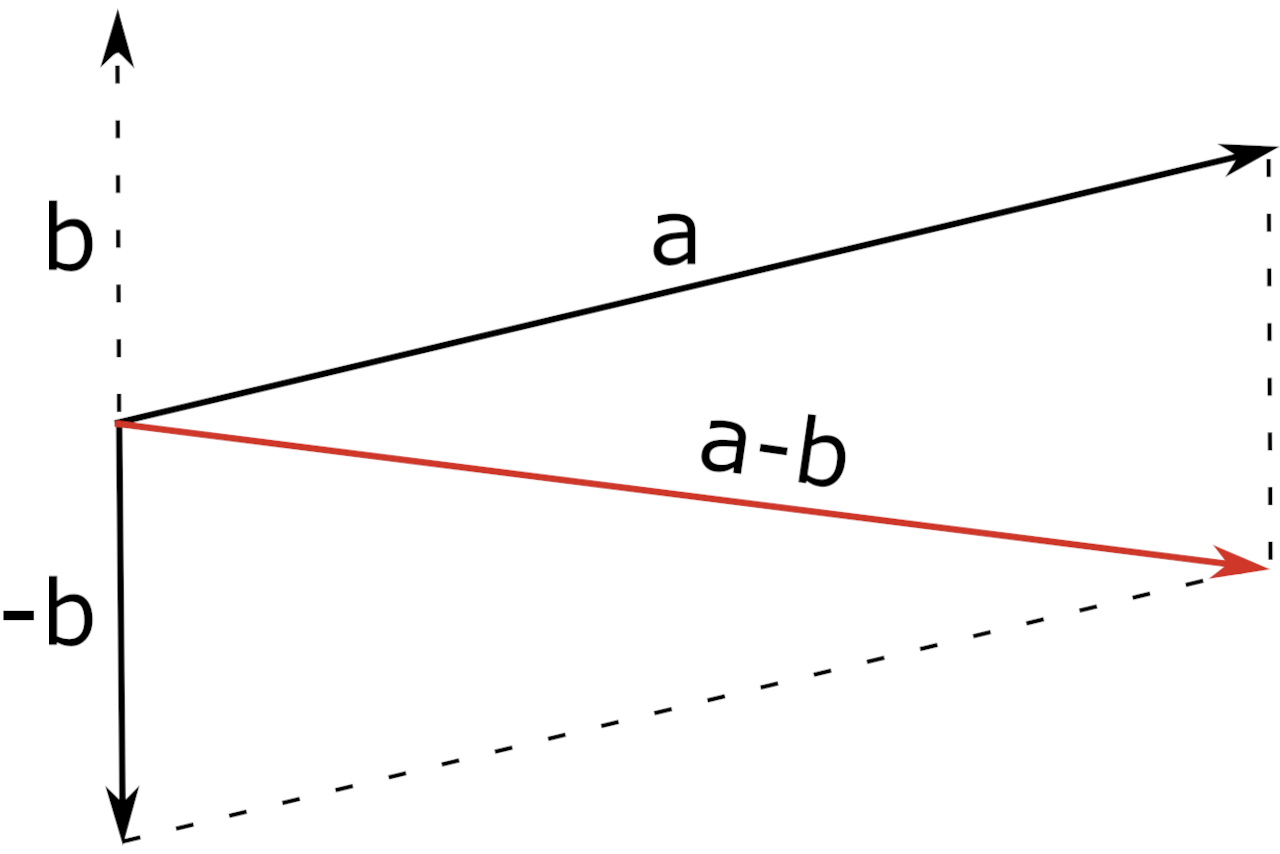# Vector Subtraction Calculator

Subtract two vectors a and b to find resultant vector a – b using the calculator below.

Vector a
Vector b
Vector a
Vector b

## Vector (a - b):

### Steps to Solve

#### Use the Vector Subtraction Formula

a - b = ({xa - xb}, {ya - yb}, {za - zb})

#### Substitute Values and Solve

Enter vectors a & b above to see the solution here

Learn how we calculated this below

## How to Subtract Vectors

In linear algebra, vectors represent ordered sequences of numbers. You can subtract vectors by subtracting the corresponding components from one another.

The difference of two vectors is referred to as the resultant vector. You can also add vectors using the same technique.

### Vector Subtraction Formula

So, the vector subtraction formula looks like this:

a – b = ({xa – xb}, {ya – yb}, {za – zb})

Thus, vector a – b is equal to the difference of the x coordinates of each vector, the difference of the y coordinates of each vector, and the difference of the z coordinates of each vector.

## Parallelogram Method

You can also subtract vectors a and b using the parallelogram method.

Using this method, place the two vectors at the same initial point. Then, complete the parallelogram by drawing two additional lines.

Because a negative vector follows the exact opposite direction of the positive vector, you can use the same method as vector addition to solve a vector subtraction problem.

a – b = a + (-b)The diagonal of the parallelogram is equal to the resultant vector a – b.

You can also use our cross product calculator or dot product calculator to multiply vectors.